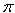Question from someone with a pool: I need to calculate how many litres in my swiming pool, it is a circle shape, 5.5 m in diametre by 1.07 m deep. Hi there. The water in a round swimming pool is in the shape of a cylinder. You can calculate the volume, v, of a cylinder with the equation v =r2 d, whereis about 3.1416, r is the radius of the pool and d is its depth. The radius is just half the diameter and you already know the depth, so you can calculate the volume with this formula. The formula gives you volume in the dimensions you use for radius and depth. Using meters for these measurements, the volume is then in meters3 . To convert that to litres, you just need to remember that there are 1000 litres in each cubic meter. So multiply by 1000. Hope this helps, Stephen La Rocque. >